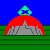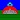思维学网

——玄易居士

【摘要】
【关键词】

【】【】【】【】【】

（1） j(t,t)=t
（2） j(t,y)=y
（3） j(y,y)=t
（4） j(y,t)=y

（1） j(t,t)=t相当于(1) 同与同相同
（2） j(t,y)=y相当于(2) 同与异相异
（3） j(y,y)=t相当于(3) 异与同相异
（4） j(y,t)=y相当于(4) 异与异相同

j(xi,xj)= xk

j（小狗，小猫） = 小狗小猫相比较

j（小麦、玉米） = 小麦玉米相比较

fh(m,ti)=xi             复合运算
fh(m,tj)=xj             复合运算
xt(xi,xj)= xt(xj ,xi)=m    析同运算
by(xi,xj)=ti ，by(xj,xi)=tj  辨异运算

fh表示复合、还原的意思，xt是析同，表示找出相同之处，by是辨异，表示找出不同之处

©2008 - 2023www.siweixue.com 鲁ICP备08003828号-1